Courses

# Test: Fluid Mechanics Level - 3

## 25 Questions MCQ Test Mechanical Engineering SSC JE (Technical) | Test: Fluid Mechanics Level - 3

Description
This mock test of Test: Fluid Mechanics Level - 3 for Mechanical Engineering helps you for every Mechanical Engineering entrance exam. This contains 25 Multiple Choice Questions for Mechanical Engineering Test: Fluid Mechanics Level - 3 (mcq) to study with solutions a complete question bank. The solved questions answers in this Test: Fluid Mechanics Level - 3 quiz give you a good mix of easy questions and tough questions. Mechanical Engineering students definitely take this Test: Fluid Mechanics Level - 3 exercise for a better result in the exam. You can find other Test: Fluid Mechanics Level - 3 extra questions, long questions & short questions for Mechanical Engineering on EduRev as well by searching above.
QUESTION: 1

Solution:
QUESTION: 2

Solution:
QUESTION: 3

### The line of action of the buoyant force acts through the centroid of the

Solution:
QUESTION: 4

The time oscillation of a floating body with increase in metacentric height will be

Solution:
QUESTION: 5

The velocity at the exit of the pipe as shown in the below figure will be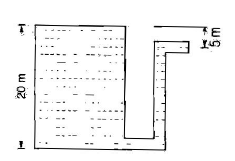Solution:
QUESTION: 6

The following terms relate to floating bodies :
Centre of gravity .....G
Meta Centre .....M
Weight of floating body.....W
Buoyant force ......FB
Match List I with List II and select these correct answer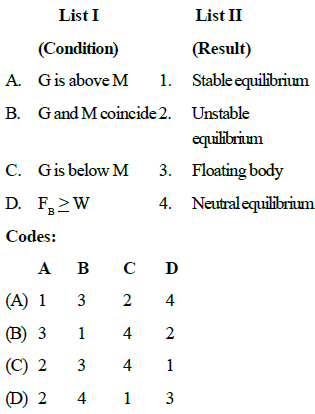Solution:
QUESTION: 7

Match List I with List II and select the correct answer.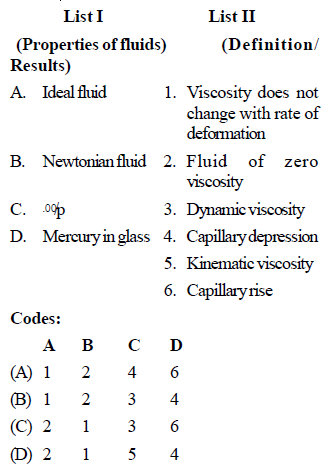Solution:

Explanation : a) A fluid that has no resistance to shear stress is known as an ideal or inviscid fluid. Zero viscosity is observed only at very low temperatures in superfluids. Otherwise, the second law of thermodynamics requires all fluids to have positive viscosity; such fluids are technically said to be viscous or viscid.

A Newtonian fluid is one whose viscosity is not affected by shear rate: all else being equal, flow speeds or shear rates do not change the viscosity.

The liquid which wets the wall of tube rises in the tube and the liquid which does not wet the wall of the tube descends in the tube. For example, when a glass capillary tube is dipped in water, water rises in the tube and the shape of water meniscus in concave, similarly when a glass capillary tube is dipped into mercury; mercury descends in the tube and the shape of mercury meniscus is convex.

QUESTION: 8

Match List I with List II and select the correct answer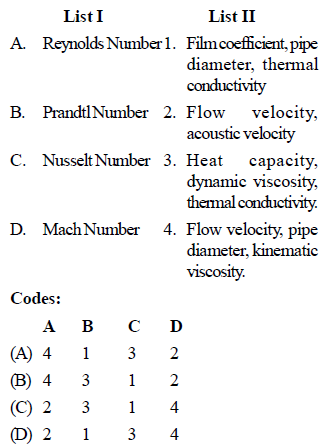Solution:
QUESTION: 9

Match List I (fluid properties) with List II (related terms) and select the correct answer.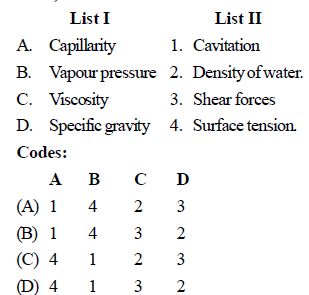Solution:
QUESTION: 10

Match List I with List II and select the correct answer.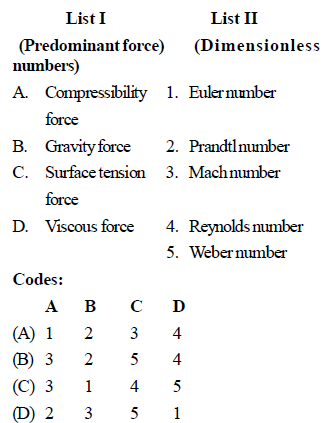Solution:
QUESTION: 11

Newton's law of viscosity for a fluid states that the shear stress is

Solution:
QUESTION: 12

A vertically immersed surface is shown in the below figure. The distance of its centre of pressure from the water surface is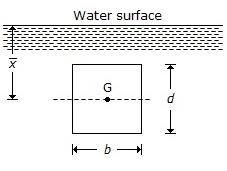Solution:
QUESTION: 13

If angle of contact of a drop of liquid is acute than

Solution:
QUESTION: 14

Match List I (Physical properties of fluid) with List II (Dimensions/definitions) and select the correct answer using the codes given below the lists: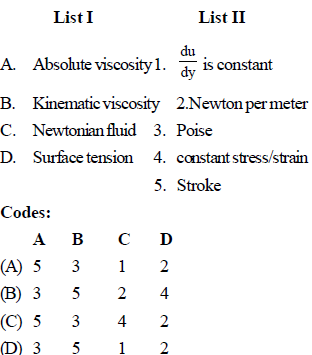Solution:
QUESTION: 15

An increase in pressure of 2 bars decreases the volume of a liquid by 0.01 percent. The bulk modulus of elasticity of the liquid is

Solution:
QUESTION: 16

The pressure inside a soap bubble of 50 mm diameter is 25 N/m2 above the atmospheric pressure. What is the surface tension is soap film

Solution:
QUESTION: 17

Bourdon gauge measures

Solution:
QUESTION: 18

When can a piezometer be not used for pressure measurement in pipes

Solution:
QUESTION: 19

How is the difference of pressure head h measured by mercury oil differential manometer expressed

where × = Manometer reading Sg and So are the specific gravities of mercury and oil respectively

Solution:
QUESTION: 20

In order to increase sensitivity of U-tube manometer, one leg is usually inclined by angle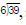sensitivity of inclined tube to sensitivity of Utube equal to

Solution:
QUESTION: 21

In figure if the pressure of gas in bulb A is 50 cm Hg vacuum and Patm = 76 cm Hg, the height of column H is equal to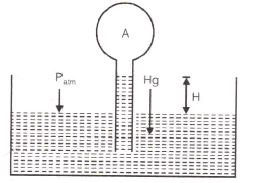Solution:
QUESTION: 22

A U-tube manometer is used to measure the pressure in an oil pipe A as shown in the figure. The specific gravity of the oil is 0.8 and that of mercury is 13.6. The equivalent gauge pressure is nearly.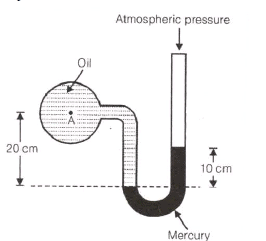Solution:
QUESTION: 23

A multi-tube manometer filled with water up to level A, B and C as shown in the figure is rotated about the vertical axis at A. The water levels at A, B and C will all lie on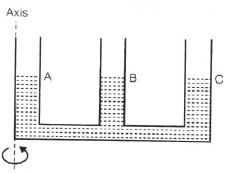Solution:
QUESTION: 24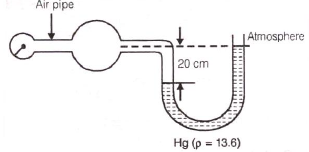A U-tube manometer shown attached to an air pipe reads a height of 20 cm of mercury as shown. What is the pressure in kPa in the air pipe ?

Solution:
QUESTION: 25

A metal block is thrown into deep lake. As it sinks deeper in water, the buoyant force acting on it

Solution: